CalculusShowing results 1 to 10 of 52, on page 1 of 6 Differentiation/Differentals Integration/Integrals Limits Sequences and Series
 DESCRIPTION EQUATION Arithmetic Series - Sequential IntegersArithmetic Series - Sequential Odd Integers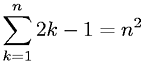Arithmetic Series - Sequential Squared Integers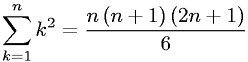Chain Rule of Differentiation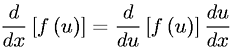Cosine Definition as an Infinite Series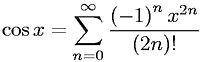Definition of a Derivative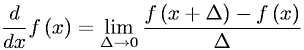Derivative of a Constant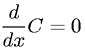Derivative of a Natural LogarithmDerivative of a Variable to the First Power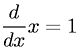Derivative of a Variable to the nth Power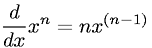Go to page: 1  2  3  4  5  6